## Monday, January 17, 2022

### Feedback and Stability

The Moon’s uneven gravity field causes most lunar orbits to be unstable. Over time the orbits increase in eccentricity, which is to say that the high part of the orbit gets higher, and the low part gets lower, until the object strikes the lunar surface. In this blog I have described the orbits of two different Apollo artifacts that show long-term stability in their orbits. (The Eagle and Snoopy.) They somehow manage to evade the instability that dooms most lunar satellites. How could that be? In this post we’ll dig in deeper to try to understand what is going on in greater detail.

I’ll start by focusing on the Eagle, and then at the end we can do a similar analysis for Snoopy. To start, as a reminder, look at the way the perilune altitude varies over time in the figure below. (Remember, perilune altitude is the lowest point of each revolution.) You see a cycle that repeats as the minimum altitude dips lower then climbs higher about every 25 days. I showed in a previous post that this 25-day cycle reflects the way the orbit changes as the Moon rotates underneath. The lowest lows always occur on the near side of the Moon. The fact that the cycle completes in 25 days, while the Moon completes a full rotation in 27.32 days, means that the Eagles orbit is also precessing. (This is also sometimes called “Apsidal advance”.) In this way the long axis of the Eagle’s orbit, called the Apse Line, does a complete circuit of the Moon in about 25 days, and this drives the short-period variation.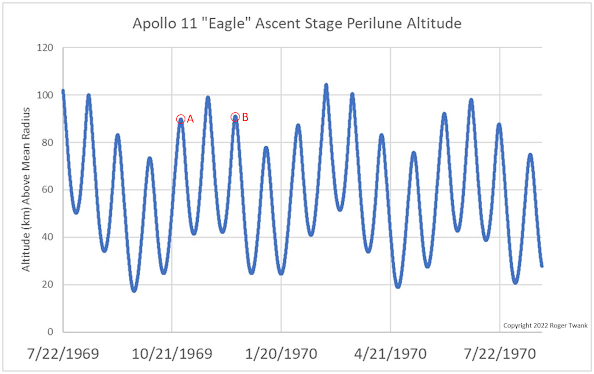Figure 1: Minimum altitude of the Eagle in the first year after jettison. Notice the shorter variations every 25 days, and the longer variation every 4-5 months.

What about that longer variation in the perilune altitude? Notice how every 4-5 months the minimum altitude goes higher and then lower. What’s going on there? If you look at the figure above, notice that the minimum altitude is nearly the same at point A and point B, but somehow this system “knows” that at point A the longer cycle is increasing, and at point B the longer cycle is decreasing. Somehow there is “state” information being stored in the system, so that it “remembers” where it is in the long-period cycle. Let’s dig in and look for that “state” signal.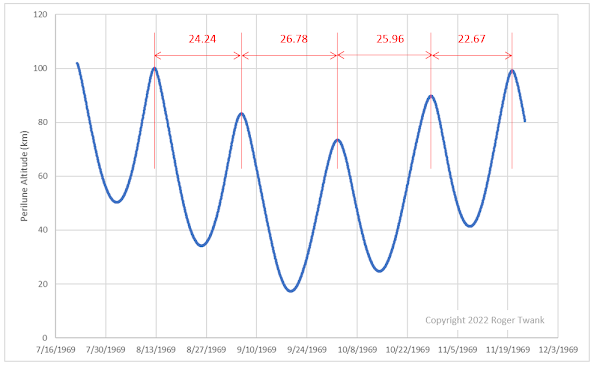Figure 2: Showing the time (in days) between the peaks of the first 4 complete cycles for the Eagle. Notice that the time between peaks increases as the altitudes move lower.

For starters, let's look for differences between the “low” cycles and “high” cycles. One thing to measure is the “period” of the cycle, i.e., how many days it takes to complete a cycle. We can measure the time between the highest point in each cycle. In figure 2 above, I show the time (in days) to go from one peak to the next for the first 4 complete cycles of the Eagle’s orbit back in 1969. Do you notice anything interesting? As the altitudes get higher, the times get a bit shorter. As the altitudes get lower, the times get a bit longer. We can plot these on a graph that makes the relationship easier to see, and in the figure below I show the first 14 cycles…the first year of the Eagle after jettison. If I plotted out the data for 52 years you would see that the same relationship continues to the present day. This is a persistent feature of the Eagle's orbit.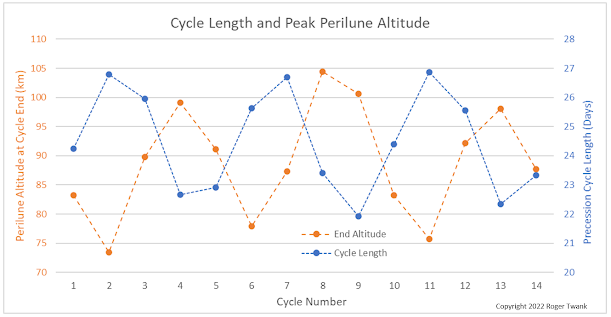Figure 3: Cycle length and end peak altitude for 14 Eagle cycles during its first year in lunar orbit.
The next thing to notice about these cycles is how they relate to the Moon. The plot below shows perilune altitude versus the Moon’s longitude, for one year. As the Moon rotates underneath the orbit, we see 14 tracks wrapping around. Each of the blue dots represents the lowest point of one revolution, and the longitude where that low point occurs above the Moon. What’s interesting is that the lowest parts of the cycles always occur on the near side of the Moon, near 30 degrees East, while the highest parts occur on the lunar far side. (From Earth we can only see lunar longitudes between -98° and +98°.)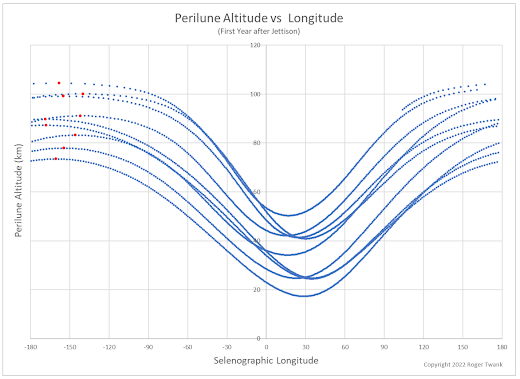Figure 4: Mapping how perilune altitude varies with lunar longitude. Eccentricity of the orbit is highest when perilune occurs on the near side of the Moon.

You might also notice some “sloshing” back and forth in that pattern in Figure 4. Notice on the left part of the figure where the highest points in each cycle are marked with red dots. The dots actually form a loop. It's even more interesting to connect the successive dots, as in Figure 5 below. In this figure I’m only showing the highest points of each cycle, like the red dots above, but now I added a dotted blue line showing the sequence. You can see that over the course of a year these dots trace out a series of loops. And these loops tie back to the slower 4- to 5-month variation you see that first figure above. Now we can see the difference between points A and B in the first figure. I’ve marked them again in Figure 5. Point A occurs about 30 degrees farther to the East than point B. This longitudinal variation is how the system stores its “state” information…how it “remembers” whether the short cycles are increasing or decreasing. And just to be clear, this is another pattern that is stable over decades. On the left in Figure 5, notice how this variation looks over a 50-year period. It doesn’t expand or contract or drift away. It remains centered on this longitude.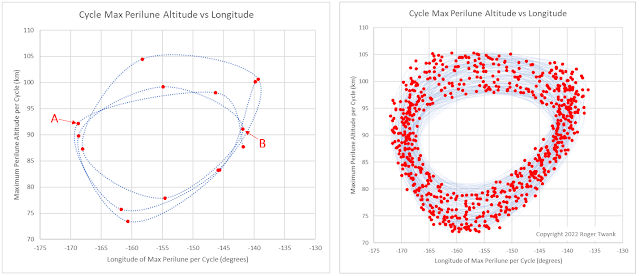Figure 5: These plots show the lunar longitude where the perilune cycle peaks occur. Points A and B on the left are the same ones marked in Figure 1. All the red points on the left are also marked in red in Figure 4. Data for 50 years is plotted on the right, showing the long-term stability of the pattern.

We’ve seen how the eccentricity variation of the orbit stays locked to lunar longitude over decades. How can that be? There must be some feedback mechanism that prevents it from drifting away. It’s interesting to look at the rate that the perilune longitude point changes. To do that, for every revolution, we have to measure how far Eastward the perilune point shifted and compare that to the elapsed time. If we divide the longitude change by the elapsed time, we get a measure of the rate. (The elapsed time is nearly constant…about 1 hour and 58 minutes per revolution, but it’s a spreadsheet doing the math, so why not recalculate it for each point.) I’ll call this measurement the “precession rate”. That’s kind of a misnomer…the longitude is mostly changing because of the Moon’s rotation under the orbit, which is not really precession. (This component is also constant, because the rate of the Moons rotation is constant.) But there is an additional precession in the orbit so there is some variation in this precession rate. Here it is...
What is interesting here is that the rate gets much faster as the perilune altitude gets higher. Put another way, the precession rate varies inversely with eccentricity. As the orbit becomes more eccentric, the rate slows down. As the rate slows down, the Moon's gravity begins to drive the eccentricity lower. Lower eccentricity causes the rate to speed up, and the cycle repeats. Again, and again. For decades.

OK we found an interesting pattern in the orbit of the Eagle that persists for decades and can plausibly explain its long-term stability. (“Explain” is a strong word here…I believe there are more layers to this onion.) How about the Snoopy descent stage? If we go through the same exercise with Snoopy, we see very similar patterns. Compare the figures below for Snoopy’s orbit to those above for the Eagle. There is something about these retrograde equatorial orbits that leads to long-term stability, somehow evading the unstable fate of most other lunar satellites. Pretty cool, eh?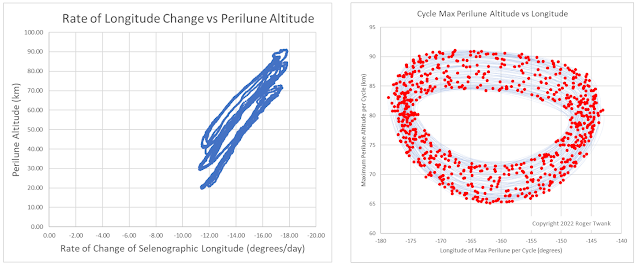Figure 7: These plots of Snoopy's orbit data show similar patterns to those of the Eagle. A similar feedback mechanism seems to be responsible for the long-term stability displayed by both orbits.